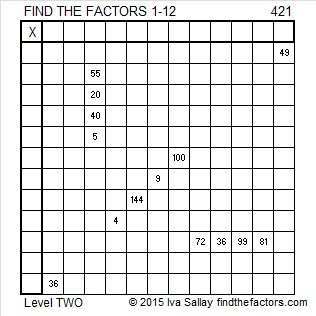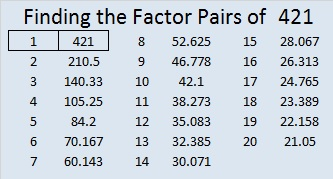# 421 and Level 2

We can easily see that 421 cannot be evenly divided by 2, 3, or 5. Could it possibly be a prime number? Scroll down to know for sure.Print the puzzles or type the factors on this excel file: 12 Factors 2015-03-09

• 421 is a prime number. 419 and 421 are twin primes.
• Prime factorization: 421 is prime.
• The exponent of prime number 421 is 1. Adding 1 to that exponent we get (1 + 1) = 2. Therefore 421 has exactly 2 factors.
• Factors of 421: 1, 421
• Factor pairs: 421 = 1 x 421
• 421 has no square factors that allow its square root to be simplified. √421 ≈ 20.5183How do we know that 421 is a prime number? If 421 were not a prime number, then it would be divisible by at least one prime number less than or equal to √421 ≈ 20.5183. Since 421 cannot be divided evenly by 2, 3, 5, 7, 11, 13, 17, or 19, we know that 421 is a prime number.This site uses Akismet to reduce spam. Learn how your comment data is processed.